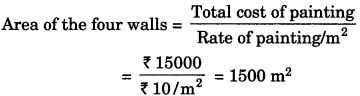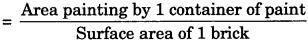# GSEB Solutions Class 9 Maths Chapter 13 Surface Areas and Volumes Ex 13.1

Gujarat Board GSEB Solutions Class 9 Maths Chapter 13 Surface Areas and Volumes Ex 13.1 Textbook Questions and Answers.

## Gujarat Board Textbook Solutions Class 9 Maths Chapter 13 Surface Areas and Volumes Ex 13.1

Question 1.
A plastic box 1.5 m long, 1.25 m wide and 65 cm deep is to be made. It is opened at the top. Ignoring the thickness of the plastic sheet, determine
1. The area of the shut required for making the box.
2. The cost of sheet for it, if a sheet measuring 1 m2 casts ₹ 20
Solution:
1. l = 1.5 m
b = 1.25 m2
and h = 65 cm = 0.65 m
∴ The area of the sheet required for making the box = lb + 2(bh + hl)
= (1.5) (1.25) + 2{(1.25) (0.65) + (0.65) (1.5)}
= 1.875 + 2{0.8125 + 0.975}
= 1.875 + 2(1.7875)
= 1.875 + 3.575
= 5.45 m2

2. The cost of sheet for it = ₹ 5.45 x 20 = ₹ 109Question 2.
The length, breadth, and height of a room are 5 m, 4 m, and 3m respectively. Find the cost of whitewashing the walls of the room and the ceiling at the rate of ₹ 7.50 perm?
Solution:
l = 5m, b = 4m and h = 3 m
Area of the walls of the room
= 2(1 + b)h = 2(5 + 4)3 = 54 m2
Area of ceiling = lb
= (5)(4) = 20 m2
Total area of the walls of the room and the ceiling
= 54 m2 + 20 m2 = 74 m2
∴ Cost of white washing the walls of the room and the ceiling
= 74 x ₹ 7.50 = ₹ 555.

Question 3.
The floor of a rectangular hall has a perimeter 250 m. If the cost of painting the four walls at the rate of ₹ 10 per m2 is ₹ 15000, find the height of the hall.
[Hint. Area of the four walls = Lateral surface area]
Solution:
Let the length, breadth, and height of the rectangular hall be l m, bm, and hm respectively.
Perimeter = 250 m
2(l + b) = 250 ⇒ l + b = 125∴ 2(l+ b)h = 1500
(l + b)h = 750
125 h = 750
h = $$\frac {750}{125}$$
h = 6m
Hence, the height of the hall is 6 m.Question 4.
The paint in a certain container is sufficient to paint on an area equal to 9.375 m2. How many bricks of dimensions 22.5 cm x 10 cm x 7.5 cm can be painted out of this container?
Solution:
For a brick, l = 22.5 cm, b = 10 cm and h = 7.5 cm
∴ Total surface area of a brick = 2(lb + bh + hl)
= 2(22.5 x 10 + 10 x 7.5 + 7.5 x 22.5)
= 2(225 + 75 + 168.75)
= 2(468.75) = 937.5 cm2 = 0.9375 m2
∴ Number of bricks that can be painted out= $$\frac {9.375}{0.9375}$$ = 10

Question 5.
A cubical box has each edge 10 cm and another cuboidal box is 12.5 cm long, 10 cm wide and 8 cm high.
1. Which box has the greater lateral surface area and by how much?
2. Which box has the smaller total surface area and by how much?
Solution:
1. Each edge of the cubical box (a) = 10 cm
∴ Lateral surface area of the cubical box
= 4a2 = 4(10)2 = 400 cm2
For cuboidal box
l = 12.5 cm, b = 10 cm and h = 8 cm
∴ Lateral surface area of the cuboidal box
= 2(l + b)h
= 2(12.5 + 10)(8) = 360 cm2
∴ Cubical box has the greater lateral surface area than the cuboidal box by (400 – 360) cm2 , i.e., 40 cm2 .2. Total surface area of the cubical box = 6a2
= 6(10)2 = 600 cm2
Total surface area of the cuboidal box
= 2 (lb + bh + hl)
= 2[(12.5) (10) + (10) (8) + (8) (12.5)]
= 2[125 + 80 + 100] = 610 cm2
∴ Cubical box has the smaller total surface area than the cuboidal box by (610 – 600) cm2, i.e., 10 cm2.

Question 6.
A small indoor greenhouse (herbarium) is made entirely of glass panes (including base) held together with tape. It is 30 cm long, 25 cm wide and 25 cm high.
1. What is the area of the glass?
2. How much of tape is needed for all the 12 edges?
Solution:
1. For herbarium
l = 30 cm
b = 25 cm
h = 25 cm
∴ Area of the glass = 2(lb + bh + hl)
= 2 [(30) (25) + (25) (25) + (25) (30)]
= 2 [750 + 625 + 750] = 4250 cm2

2. The tape needed for all the 12 edges
= 4 (l + b + h)
= 4(30 + 25 + 25)
= 320 cmQuestion 7.
Shanti Sweets Stall was placing an order for making cardboard boxes for packing their sweets. Two sizes of boxes were required. The bigger of dimensions 25 cm x 20 cm x 5 cm and the smaller of dimensions 15 cm x 12 cm x 5 cm. For all the overlaps, 5% of the total surface area is required extra. If the cost of the cardboard is ₹ 4 for 1000 cm2, find the cost of cardboard required for supplying 250 boxes of each kind.
Solution:
For bigger box
l = 25 cm
b = 20 cm
h = 5 cm
∴ Total surface area of the bigger box = 2 (lb + bh + hi)
= 2[(25) (20) + (20) (5) + (5) (25)]
= 2 [500 + 100 + 125] = 1450 cm2 Cardboard required for all the overlaps
= 1450 x $$\frac {5}{100}$$ = 72.5 cm2
∴ Net surface area of the bigger box = 1450 cm2 + 72.5 cm2 = 1522.5 cm2
∴ Net surface area of 250 bigger boxes = 1522.5 x 250 = 380625 cm2
∴ Cost of cardboard
= $$\frac {4}{1000}$$ x 380625 = ₹ 1522.50
For smaller box
l = 15 cm
b = 12 cm
h = 5 cm
∴Total surface area of the smaller box
= 2 (lb + bh + hl)
= 2[(15) (12) + (12) (5) + (5) (15)]
= 2 [180 + 60 + 75] = 630 cm2Cardboard required for all the overlaps
= 630 x $$\frac {5}{100}$$ = 31.5 cm2
∴ Net surface area of the smaller box
= 630 cm2 + 31.5 cm2 = 661.5 cm2
∴ Net surface area of 250 smaller boxes
= 661.5 x 250 = 165375 cm2
Cost of cardboard
$$\frac {4}{1000}$$ x 165375 = ₹ 661.50
∴ Cost of cardboard required for supplying 250 boxes of each kind
= ₹ 1522.50 + ₹ 661.50 = ₹ 2184

Question 8.
Parveen wanted to make a temporary shelter for her car, by making a box-like structure with a tarpaulin that covers all the four sides and the top of the car (with the front face as a flap which can be rolled up). Assuming that the stitching margins are very small and therefore negligible, how many tarpaulins would be required to make the shelter of height 2.5 m, with base dimensions 4 m x 3 m?
Sol. For shelter
l = 4 m
b = 3 m
h = 2.5 m
∴ Total surface area of the shelter
= lb + 2(bh + hl)
= (4) (3) + 2[(3) (2.5) + (2.5) (4)]
= 12 + 2 [7.5 + 10] = 47 m2
Hence, 47 m2 of tarpaulin will be required.